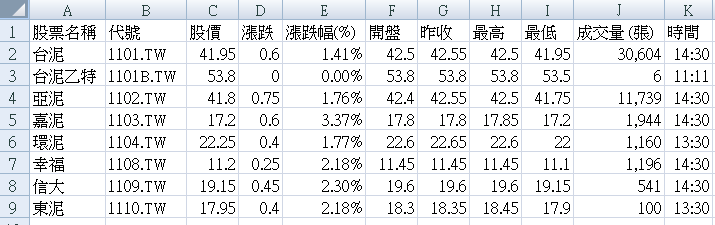## 2021年5月23日 星期日

### [疫情學習不中斷]應學員要求整理最近VBA與Python教學課程

[疫情學習]應學員要求整理最近VBA與Python教學課程

EXCEL VBA入門+進階合輯2020(光碟1-4) NT\$3500元
PYTHON合輯2020(光碟5-8) NT\$3500元
EXCEL VBA入門+進階+PYTHON合輯2020(光碟1-8) NT\$6000元

2021版：gg.gg/DVD_apply2021

## 2021年5月22日 星期六

### 用EXCEL VBA網路爬蟲擷取YAHOO股市資料

EXCEL錄製巨集爬取YAHOO股市資料，

https://tw.stock.yahoo.com/class-quote?sectorId=1&exchange=TAI

# *改用IE物件下載## VBA程式碼

Sub 下載YAHOO_水泥()

'清除資料

Cells.Clear

'1.建立IE物件

Set ie = CreateObject("internetexplorer.application")

'2.連線到網址

ie.navigate "https://tw.stock.yahoo.com/class-quote?sectorId=1&exchange=TAI"

'3.等待3秒

Application.Wait (Now + TimeValue("0:00:5"))

'4.取得網頁中第1個表格

Set tbl = ie.document.getElementsByTagName("ul")(5)

'5.取得表格中所有的列<tr>

Set trs = tbl.getElementsByTagName("li")

'    '6.取得列中的每一個儲存格內文字<td>如果沒有<td>抓<th>

For i = 0 To trs.Length - 1

Set tds = trs(i).getElementsByTagName("div")

For j = 5 To tds.Length - 1

Cells(i + 1, j - 4) = tds(j).innertext

Next

Next

Columns("C").Delete

ie.Quit

Rows(1).Insert

Range("A1") = "股票名稱": Range("B1") = "代號": Range("C1") = "股價"

Range("D1") = "漲跌": Range("E1") = "漲跌幅(%)": Range("F1") = "開盤"

Range("G1") = "昨收": Range("H1") = "最高": Range("I1") = "最低"

Range("J1") = "成交量 (張)": Range("K1") = "時間"

Columns.AutoFit

End Sub

# 什麼是IE物件：

https://msdn.microsoft.com/en-us/library/aa752084(v=vs.85).aspx

EXCEL VBA進階班的課程規劃

 函數東吳進修推廣部, EXCEL, EXCEL VBA 函數,程式設計,線上教學